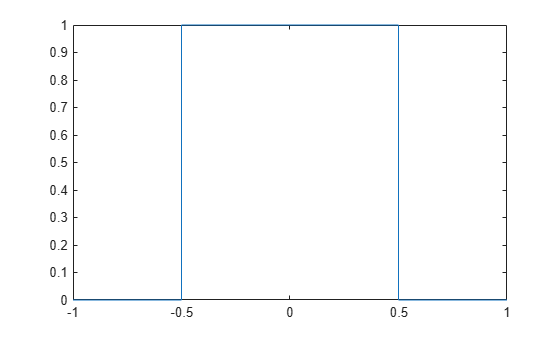Main Content

# rectangularPulse

Rectangular pulse function

## Syntax

``rectangularPulse(a,b,x)``
``rectangularPulse(x)``

## Description

example

````rectangularPulse(a,b,x)` returns the Rectangular Pulse Function.```
````rectangularPulse(x)` is a shortcut for `rectangularPulse(-1/2,1/2,x)`.```

## Examples

collapse all

Plot the rectangular pulse function using `fplot`.

```syms x fplot(rectangularPulse(x), [-1 1])```Compute the rectangular pulse function for these numbers. Because these numbers are not symbolic objects, you get floating-point results.

```[rectangularPulse(-1, 1, -2) rectangularPulse(-1, 1, -1) rectangularPulse(-1, 1, 0) rectangularPulse(-1, 1, 1) rectangularPulse(-1, 1, 2)]```
```ans = 0 0.5000 1.0000 0.5000 0```

Compute the rectangular pulse function for the same numbers in symbolic form.

```[rectangularPulse(sym(-1), 1, -2) rectangularPulse(-1, sym(1), -1) rectangularPulse(-1, 1, sym(0)) rectangularPulse(sym(-1), 1, 1) rectangularPulse(sym(-1), 1, 2)]```
```ans = 0 1/2 1 1/2 0```

Show that if `a < b`, the rectangular pulse function for `x = a` and `x = b` equals `1/2`.

```syms a b x assume(a < b) rectangularPulse(a, b, a) rectangularPulse(a, b, b)```
```ans = 1/2 ans = 1/2```

For further computations, remove the assumptions on the variables by recreating them using `syms`:

`syms a b`

For `a = b`, the rectangular pulse function returns `0`:

```syms a x rectangularPulse(a, a, x)```
```ans = 0```

Compute a rectangular pulse of width by using `rectangularPulse(x)`. This call is equal to `rectangularPulse(-1/2, 1/2, x)`.

```syms x rectangularPulse(x)```
```ans = rectangularPulse(-1/2, 1/2, x)```
```[rectangularPulse(sym(-1)) rectangularPulse(sym(-1/2)) rectangularPulse(sym(0)) rectangularPulse(sym(1/2)) rectangularPulse(sym(1))]```
```ans = 0 1/2 1 1/2 0```

When the rising or falling edge of `rectangularPulse` is `Inf`, then the result is in terms of `heaviside`.

```syms x rectangularPulse(-inf, 0, x) rectangularPulse(0, inf, x) rectangularPulse(-inf, inf, x)```
```ans = heaviside(-x) ans = heaviside(x) ans = 1```

## Input Arguments

collapse all

Input, specified as a number or a symbolic scalar. This argument specifies the rising edge of the rectangular pulse function.

Input, specified as a number or a symbolic scalar. This argument specifies the falling edge of the rectangular pulse function.

Input, specified as a number, vector, matrix, or array, or a symbolic number, variable, array, function, or expression.

## More About

collapse all

### Rectangular Pulse Function

• If `a < x < b`, then the rectangular pulse function equals 1.

• If `x = a` or `x = b` and ```a <> b```, then the rectangular pulse function equals 1/2.

• Otherwise, it equals 0.

The rectangular pulse function is also called the rectangle function, boxcar function, Pi function, or gate function.

## Tips

• If `a` and `b` are variables or expressions with variables, `rectangularPulse` assumes that `a < b`. If `a` and `b` are numerical values, such that `a > b`, `rectangularPulse` throws an error.

• If `a = b`, `rectangularPulse` returns 0.

## See Also

Introduced in R2012b

## Support

#### Mathematical Modeling with Symbolic Math Toolbox

Get examples and videos